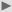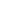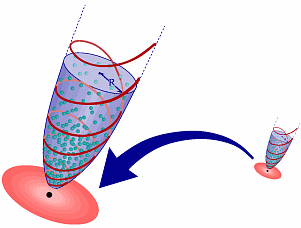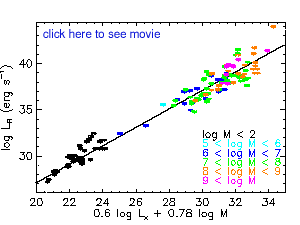R E S E A R C H   H I G H L I G H T S A U G U S T   2 0 0 3D E U T S C H E   V E R S I O N## A Jet is a Jet, Big or Small: Scale Invariance of Black Hole Jets

 Scientists at the Max-Planck-Institute for Astrophysics have found the formula that governs the emission from jets launched by black holes of different masses and different feeding rates. Their predictions have been confirmed by the subsequent discovery of a tight correlation between the radio emission, the X-ray emission, and the mass of active black holes.Figure 1: Artist's impression of black hole - accretion disk - jet systems in galactic nuclei and X-ray binaries. The appearance of the inner regions around the black hole is very similar in both cases, but one is a billion times smaller than the other.Figure 2: Schematic of the scale invariance hypothesis: To obtain the properties of a jet from a big black hole (e.g., the magnetic field strength, shown as red lines, and the density of relativistic particles, shown as green dots) it is enough to know them for a small black hole and scale them according to the ratio of their masses.Figure 3: A view of the fundamental plane of black hole activity. Plotted are the radio luminosity LR and a combination of black hole mass M and X-ray luminosity LX, such that the plane is seen exactly side-on (in other words: the measured correlation coefficients are 0.6 and 0.78, as shown on the x-axis). The colors represent different mass ranges. To view an animation of the fundamental plane, please click on the image.Objects as diverse as X-ray binaries, radio galaxies, quasars, and even our Galactic center, are powered by the gravitational energy released when surrounding gas is sucked into the black hole sitting in their cores, a process astronomers call accretion. Apart from copious radiation, one of the manifestations of this accretion energy release is the production of so-called jets, collimated beams of matter that are expelled from the innermost regions of accretion disks. These jets shine particularly brightly at radio frequencies.

The complicated physics of jet acceleration and collimation close to a black hole is still a mystery. Yet, the observed similarity (in morphology and spectrum) of jets from black holes of different mass suggests that they share a common physical origin. Scientists from the Max-Planck-Institut for Astrophysics have followed this lead and investigated the consequences of considering all astrophysical black holes to be members of one single class. They formulated a scale invariance hypothesis, which states (when translated into words) that a black hole of a billion solar masses in the nucleus of a galaxy, surrounded by a large accretion disk and launching a huge jet into the intergalactic space, can be scaled down by a factor of a billion and look exactly like a black hole of one solar mass, with its small accretion disk and jet, like the ones we observe in binary systems in our own Galaxy (see Figures 1 and 2).

They were then able to derive the underlying mathematical relation between the emission from jets, the mass of the black hole, and the rate at which matter is accreted onto it, independent of the complicated and uncertain nature of jets. Starting from the physical conditions in the accretion disk (such as the magnetic field strength and particle density, see Figure 2), it is then possible to predict how bright a jet from a black hole of given mass and accretion rate will be.

### Testing the predictions of the scale invariance hypothesis:

In an effort to test these theoretical predictions, scientists at the Max-Planck-Institute for Astrophysics have selected a sample of black holes of the most diverse kinds (according to the standard classification), spanning a range in black hole mass from several times the mass of the sun to several billion times that mass, and containing measurements both in X-rays (probing the highly energetic accretion disc emission) and at radio frequencies (probing the jet emission).

In a stunning confirmation of the scale invariance hypothesis, they discovered that all the active black holes in the sample followed a simple linear correlation that the scientists called "fundamental plane" of black hole activity. That is, if one plots the mass, radio luminosity, and X-ray luminosity for every black hole in their sample, all the plotted points will lie in a well defined plane (see Figure 3). The existence and the specific orientation of this plane (expressed in terms of the so-called "correlation coefficients") agree very well with the theoretical predictions.

The scientists have further demonstrated how a direct comparison of their theoretical predictions for the correlation coefficients with more precise future observations can test and rule out different accretion disk models and how it can measure the coupling between disks and jets in these most extraordinary and powerful engines of the Universe.R E S E A R C H   H I G H L I G H T SM P A   H O M E P A G ED E U T S C H E   V E R S I O N